Plots mathematical expressions in one and two variables.

plotFun(
object,
...,
plot = trellis.last.object(),
under = FALSE,
xlim = NULL,
ylim = NULL,
npts = NULL,
ylab = NULL,
xlab = NULL,
zlab = NULL,
filled = TRUE,
levels = NULL,
nlevels = 10,
labels = TRUE,
surface = FALSE,
groups = NULL,
col = trellis.par.get("superpose.line")$col, col.regions = topo.colors, type = "l", lwd = trellis.par.get("superpose.line")$lwd,
lty = trellis.par.get("superpose.line")$lty, alpha = NULL, discontinuities = NULL, discontinuity = 1, interactive = rstudio_is_available() ) ## Arguments object a mathematical expression or a function "of one variable" which will converted to something intuitively equivalent to object(x) ~ x. (See examples) ... additional parameters, typically processed by lattice functions such as lattice::xyplot(), lattice::levelplot() or their panel functions. Frequently used parameters include main main title for plot sub subtitle for plot lwd line width lty line type col a color or a (small) integer indicating which color in the current color scheme is desired. Additionally, these arguments can be used to specify parameters for the function being plotted and to specify the plotting window with natural names. See the examples for such usage. plot a trellis object; by default, the most recently created trellis plot. When add is TRUE, the new function will be plotted into a layer added to this object. add if TRUE, then add a layer to an existing plot rather than creating a new plot. If NULL, this will be determined by the value of under. under if TRUE, then new layer is added beneath existing layers xlim limits for x axis (or use variable names, see examples) ylim limits for y axis (or use variable names, see examples) npts number of points for plotting. ylab label for y axis xlab label for x axis zlab label for z axis (when in surface-plot mode) filled fill with color between the contours (TRUE by default) levels levels at which to draw contours nlevels number of contours to draw (if levels not specified) labels if FALSE, don't label contours surface draw a surface plot rather than a contour plot groups grouping argument ala lattice graphics col vector of colors for line graphs and contours col.regions a vector of colors or a function (topo.colors by default) for generating such type type of plot ("l" by default) lwd vector of line widths for line graphs lty vector of line types for line graphs alpha number from 0 (transparent) to 1 (opaque) for the fill colors discontinuities a vector of input values at which a function is discontinuous or NULL to use a heuristic to auto-detect. discontinuity a positive number determining how sensitive the plot is to potential discontinuity. Larger values result in less sensitivity. The default is 1. Use discontinuity = Inf to disable discontinuity detection. Discontinuity detection uses a crude numerical heuristic and may not give the desired results in all cases. interactive a logical indicating whether the surface plot should be interactive. ## Value a trellis object ## Details makes plots of mathematical expressions using the formula syntax. Will draw both line plots and contour/surface plots (for functions of two variables). In RStudio, the surface plot comes with sliders to set orientation. If the colors in filled surface plots are too blocky, increase npts beyond the default of 50, though npts=300 is as much as you're likely to ever need. See examples for overplotting a constraint function on an objective function. ## Examples plotFun( a*sin(x^2)~x, xlim=range(-5,5), a=2 ) # setting parameter value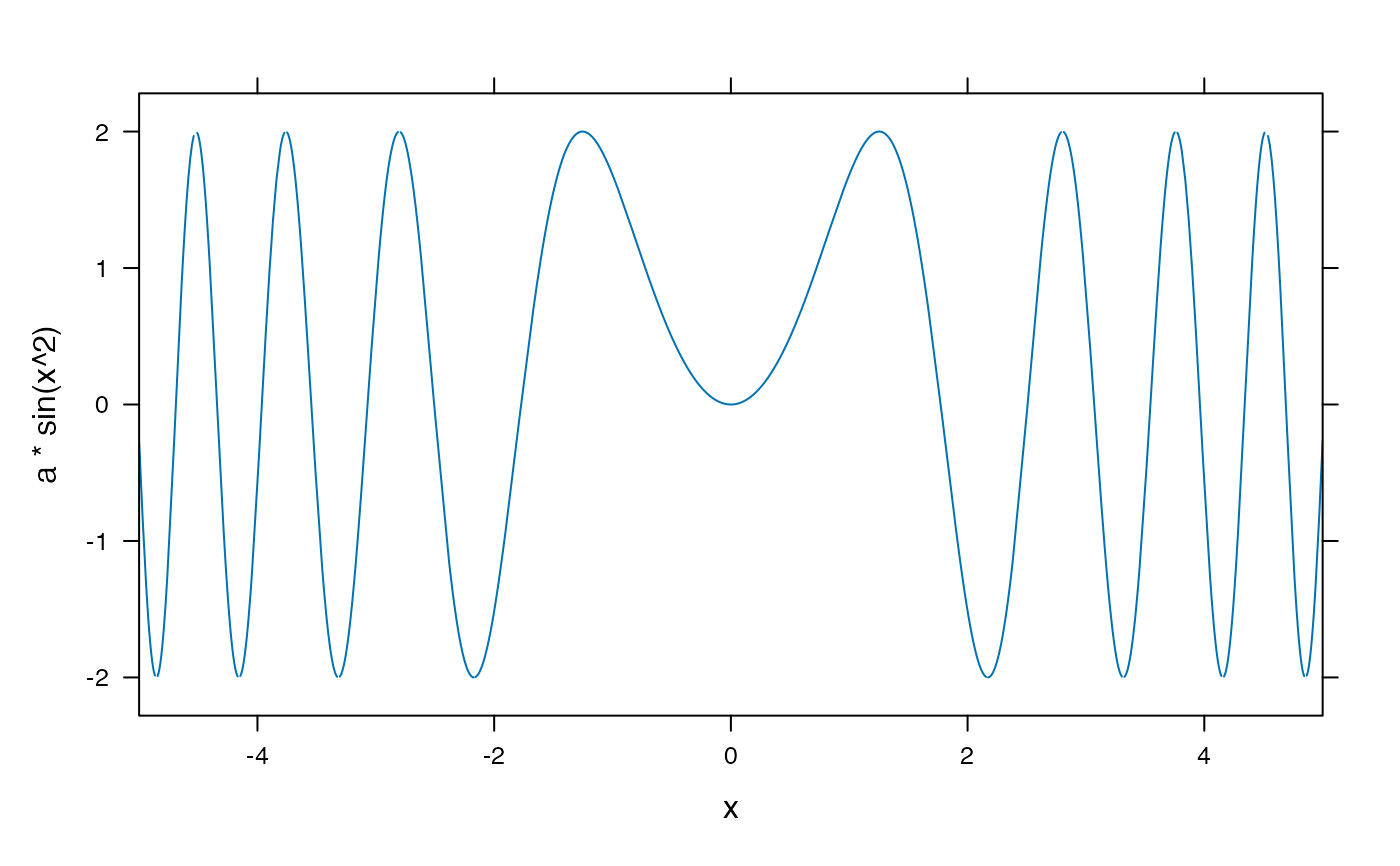plotFun( u^2 ~ u, ulim=c(-4,4) ) # limits in terms of u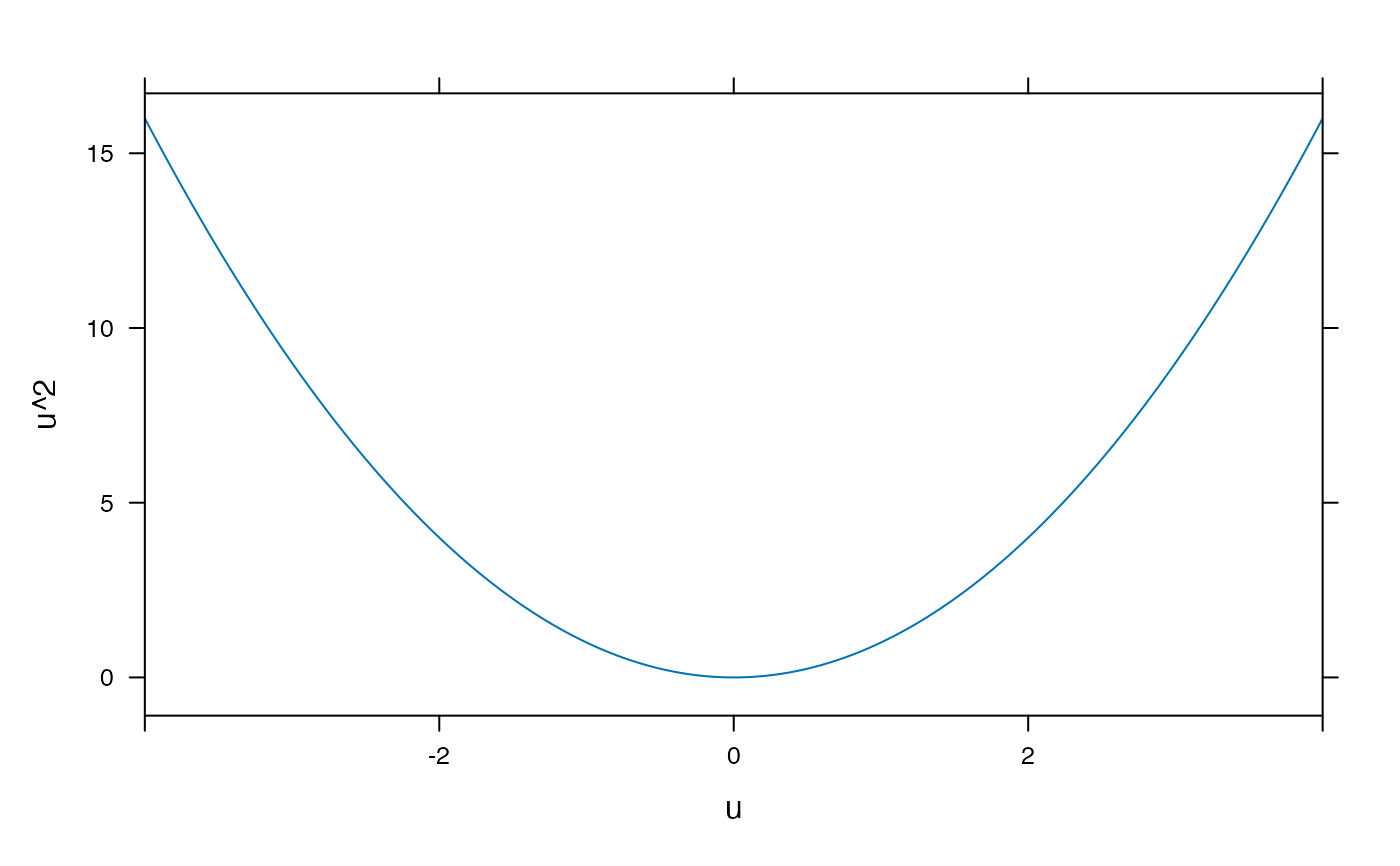# Note roles of ylim and y.lim in this example plotFun( y^2 ~ y, ylim=c(-2,20), y.lim=c(-4,4) )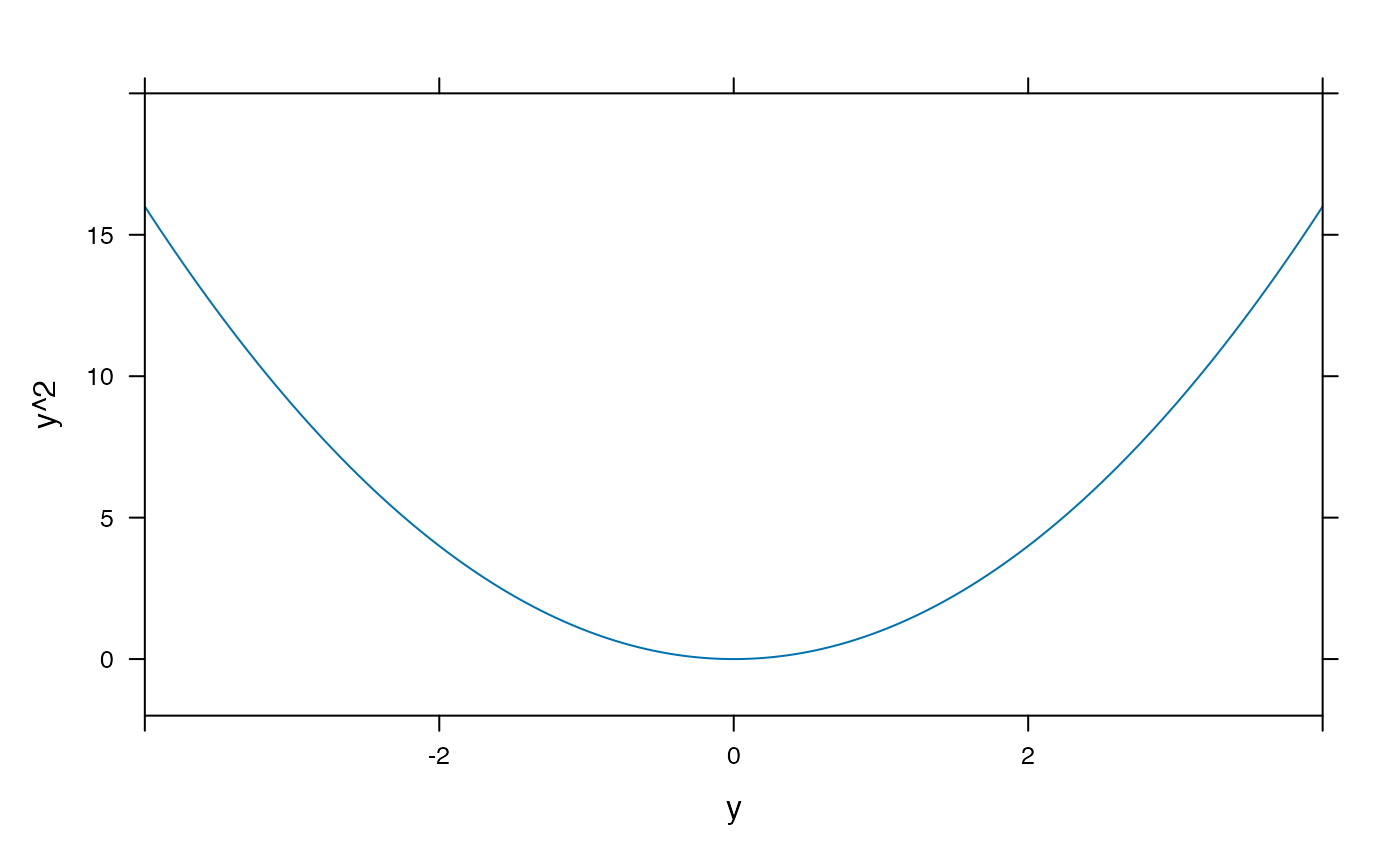# Combining plot elements to show the solution to an inequality plotFun( x^2 -3 ~ x, xlim=c(-4,4), grid=TRUE )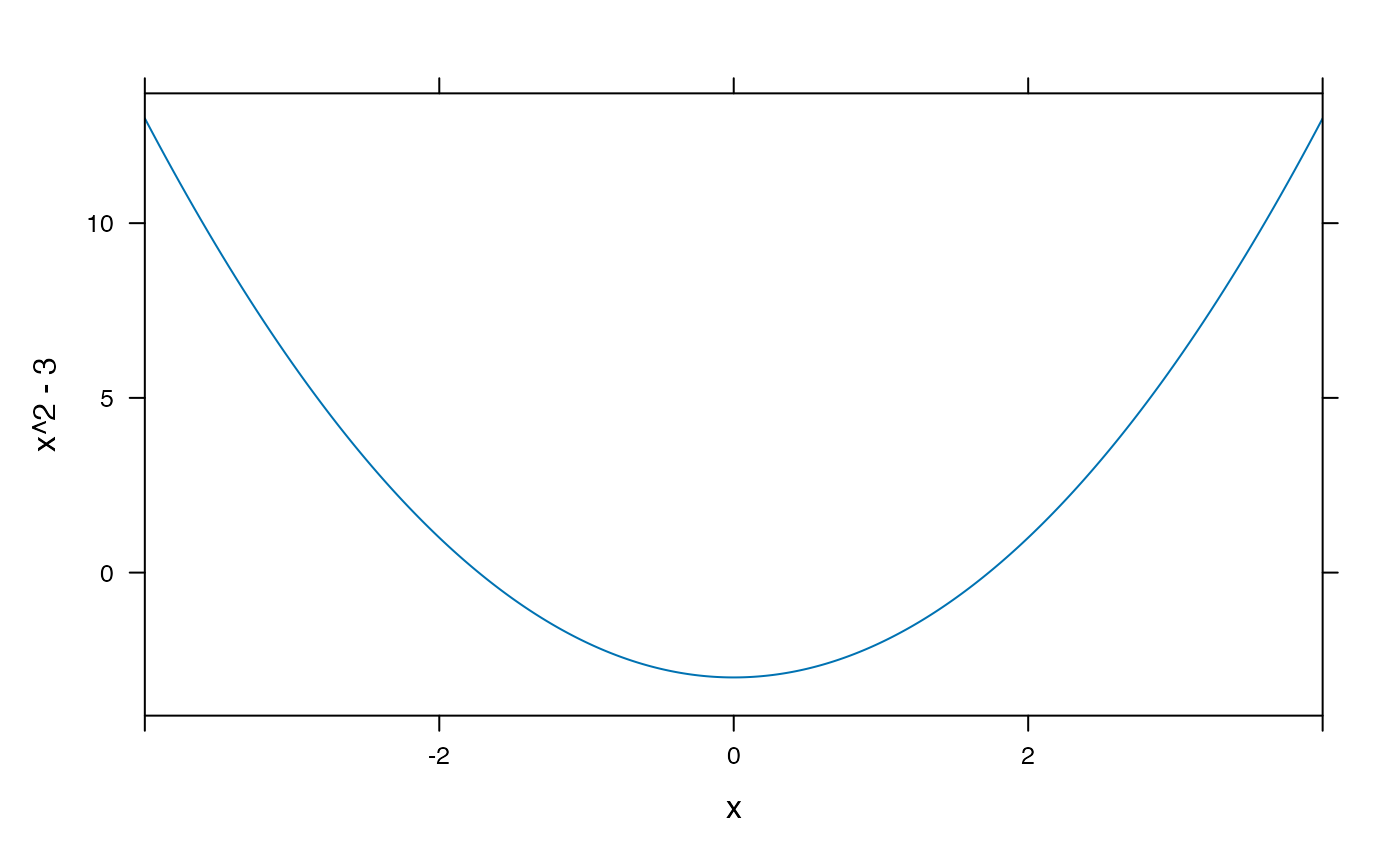ladd( panel.abline(h=0,v=0,col='gray50') )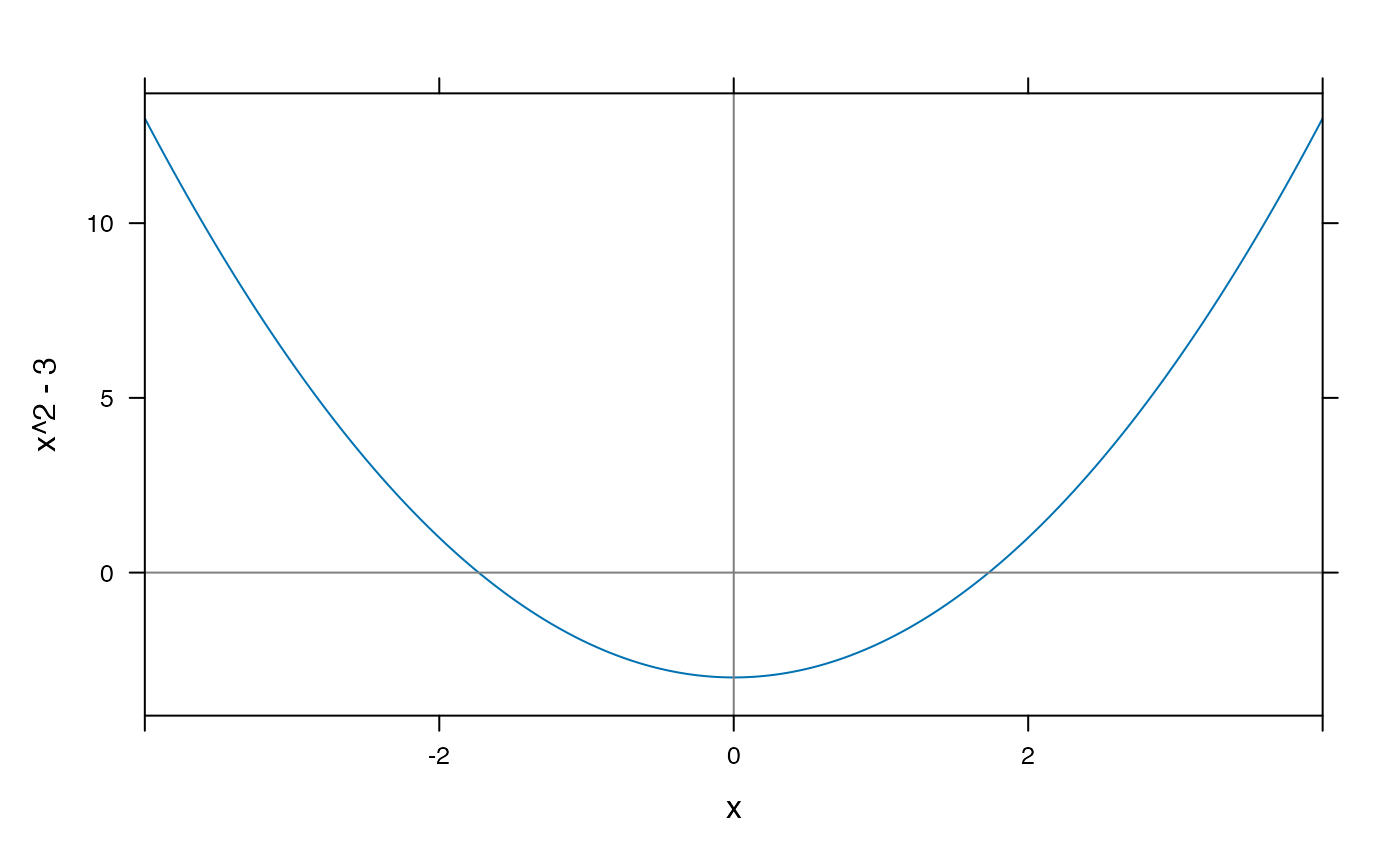plotFun( (x^2 -3) * (x^2 > 3) ~ x, type='h', alpha=.1, lwd=4, col='lightblue', add=TRUE )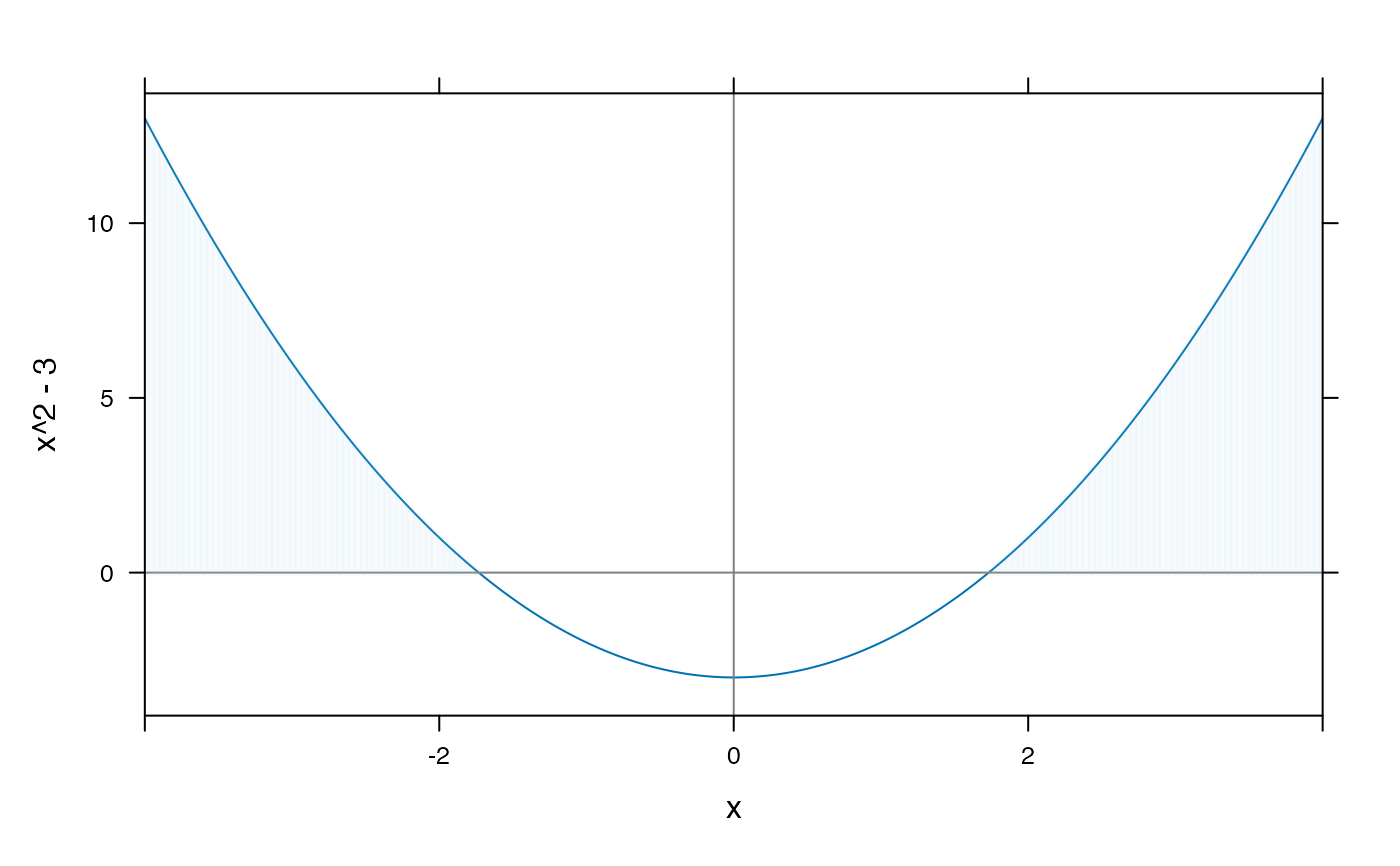plotFun( sin(x) ~ x, groups=cut(x, findZeros(sin(x) ~ x, within=10)$x),
col=c('blue','green'), lty=2, lwd=3, xlim=c(-10,10) )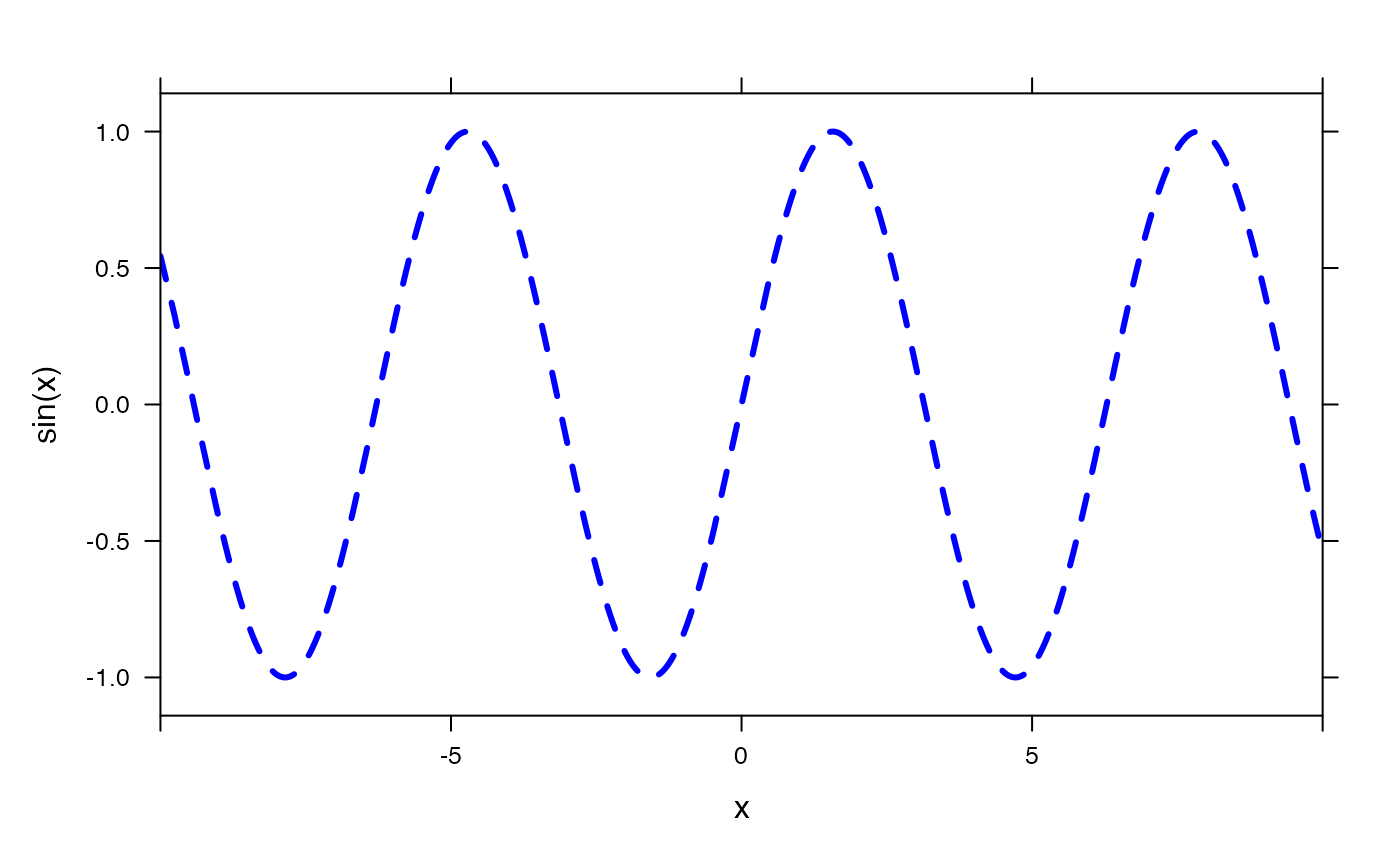plotFun( sin(x) ~ x,
groups=cut(x, findZeros(sin(x) ~ x, within=10)\$x),
col=c(1,2), lty=2, lwd=3, xlim=c(-10,10) )
#> converting numerical color value into a color using lattice settings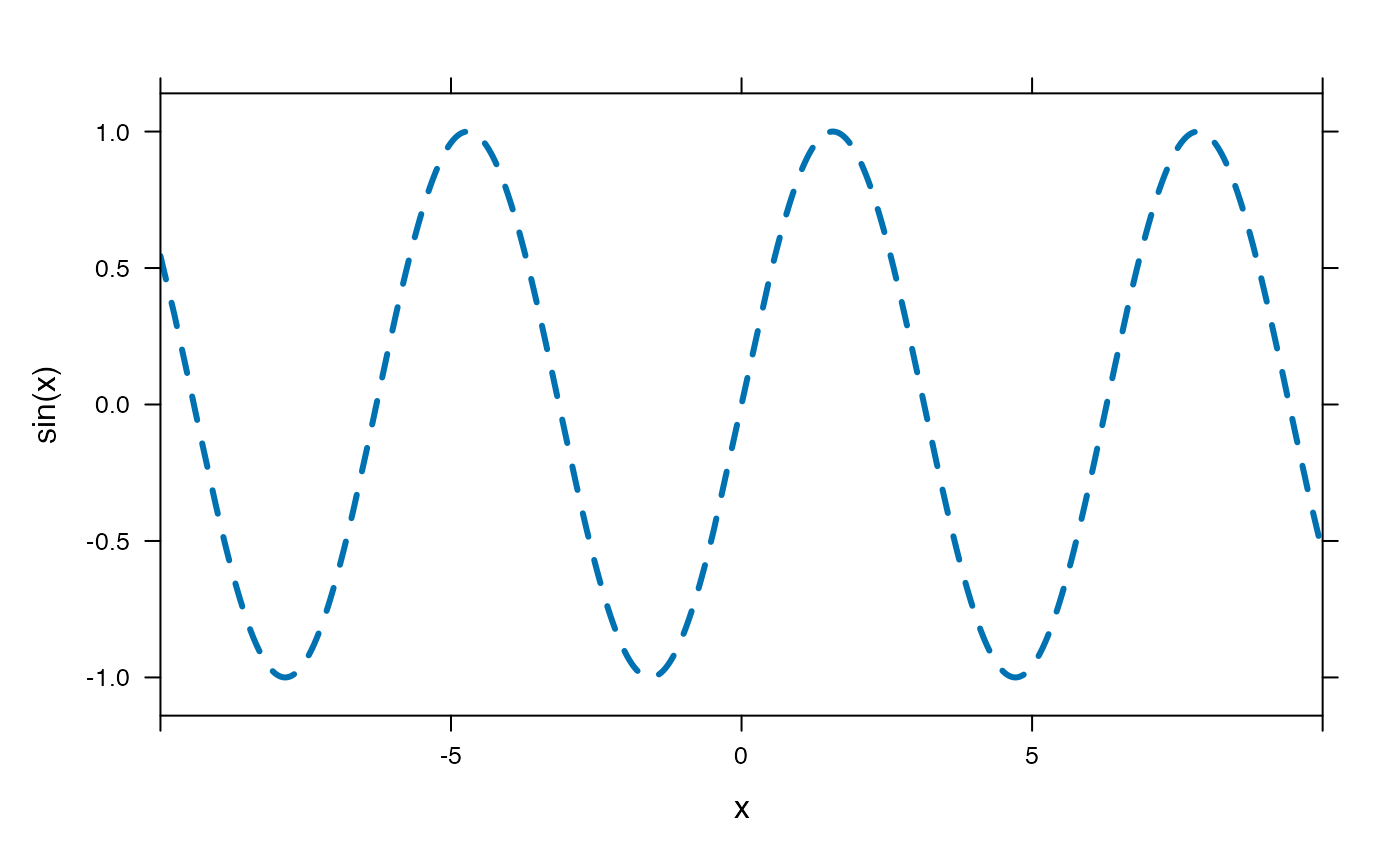## plotFun( sin(2*pi*x/P)*exp(-k*t)~x+t, k=2, P=.3)
f <- rfun( ~ u & v )
plotFun( f(u=u,v=v) ~ u & v, u.lim=range(-3,3), v.lim=range(-3,3) )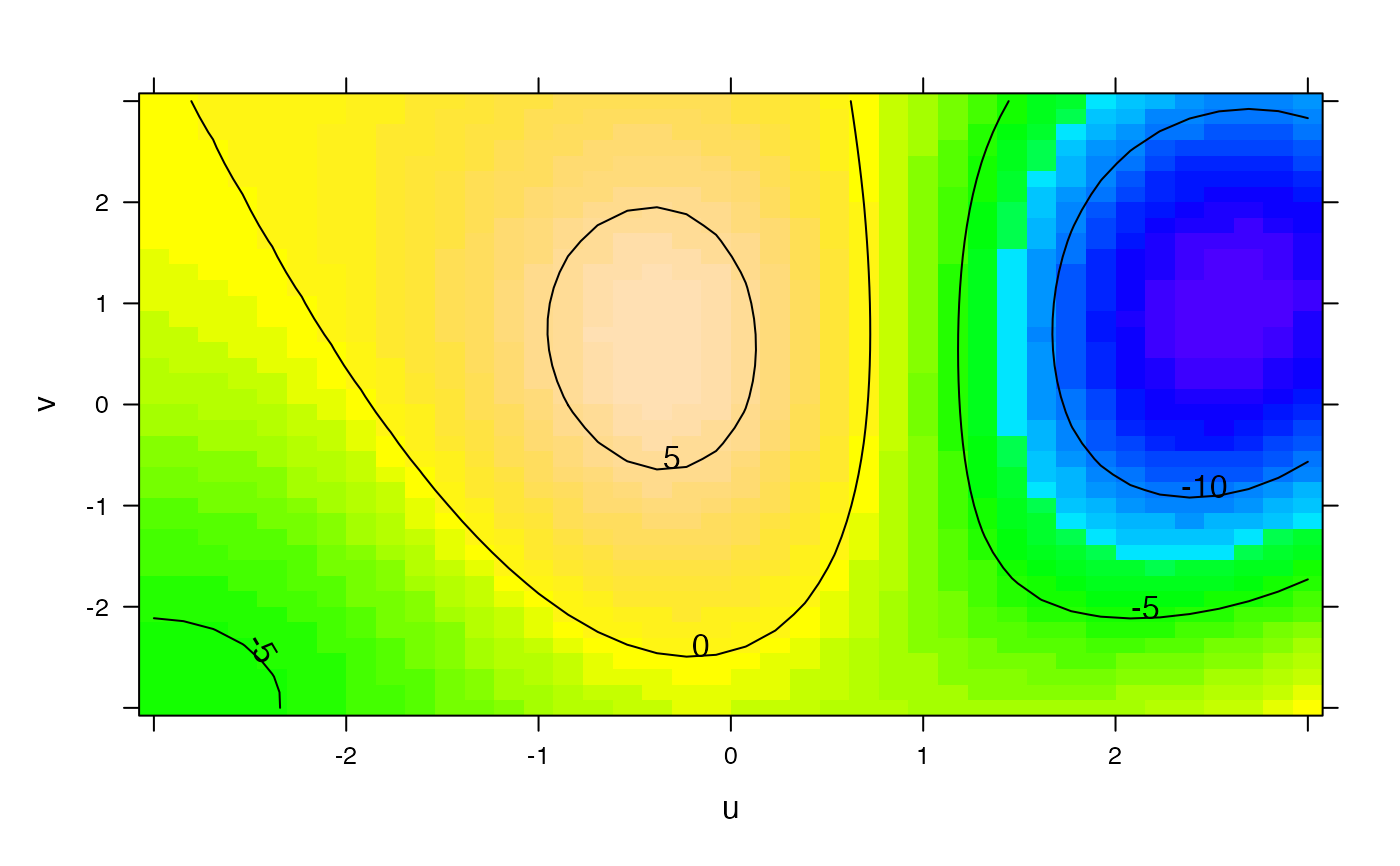plotFun( u^2 + v < 3 ~ u & v, add=TRUE, npts=200 )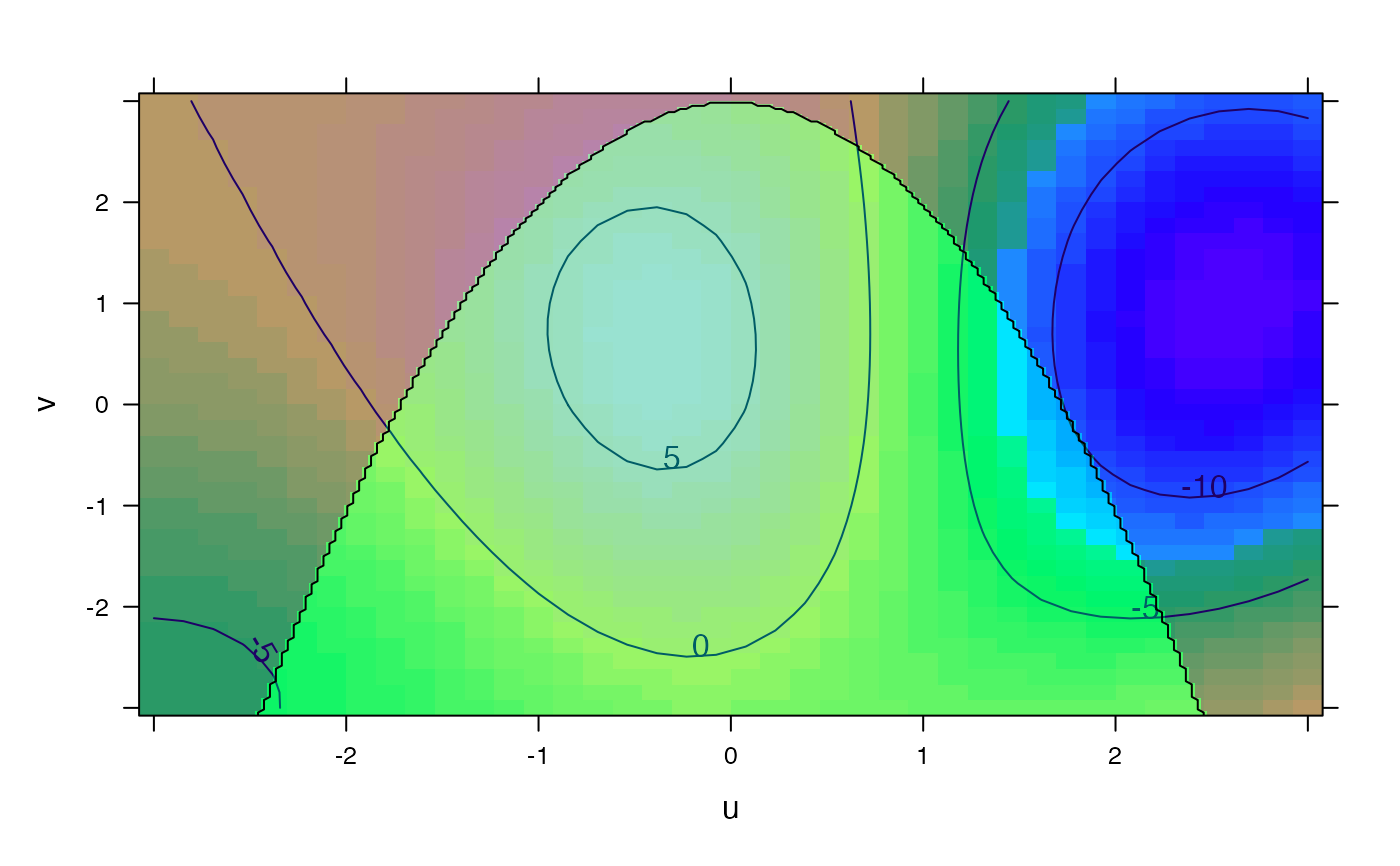if (require(mosaicData)) {
# display a linear model using a formula interface
model <- lm(wage ~ poly(exper,degree=2), data=CPS85)
fit <- makeFun(model)
xyplot(wage ~ exper, data=CPS85)
plotFun(fit(exper) ~ exper, add=TRUE, lwd=3, col="red")
# Can also just give fit since it is a "function of one variable"
}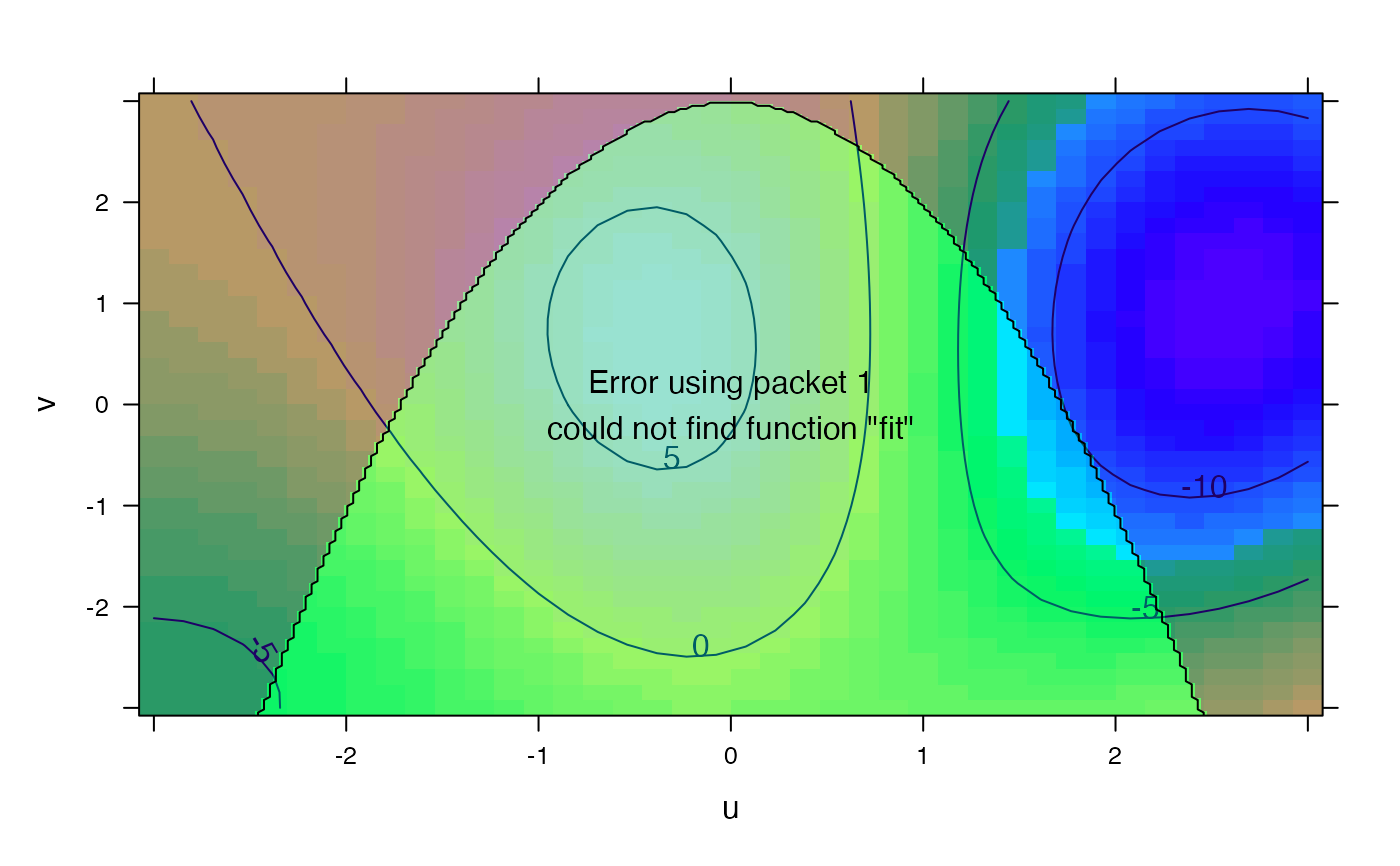# Attempts to find sensible axis limits by default
plotFun( sin(k*x)~x, k=0.01 )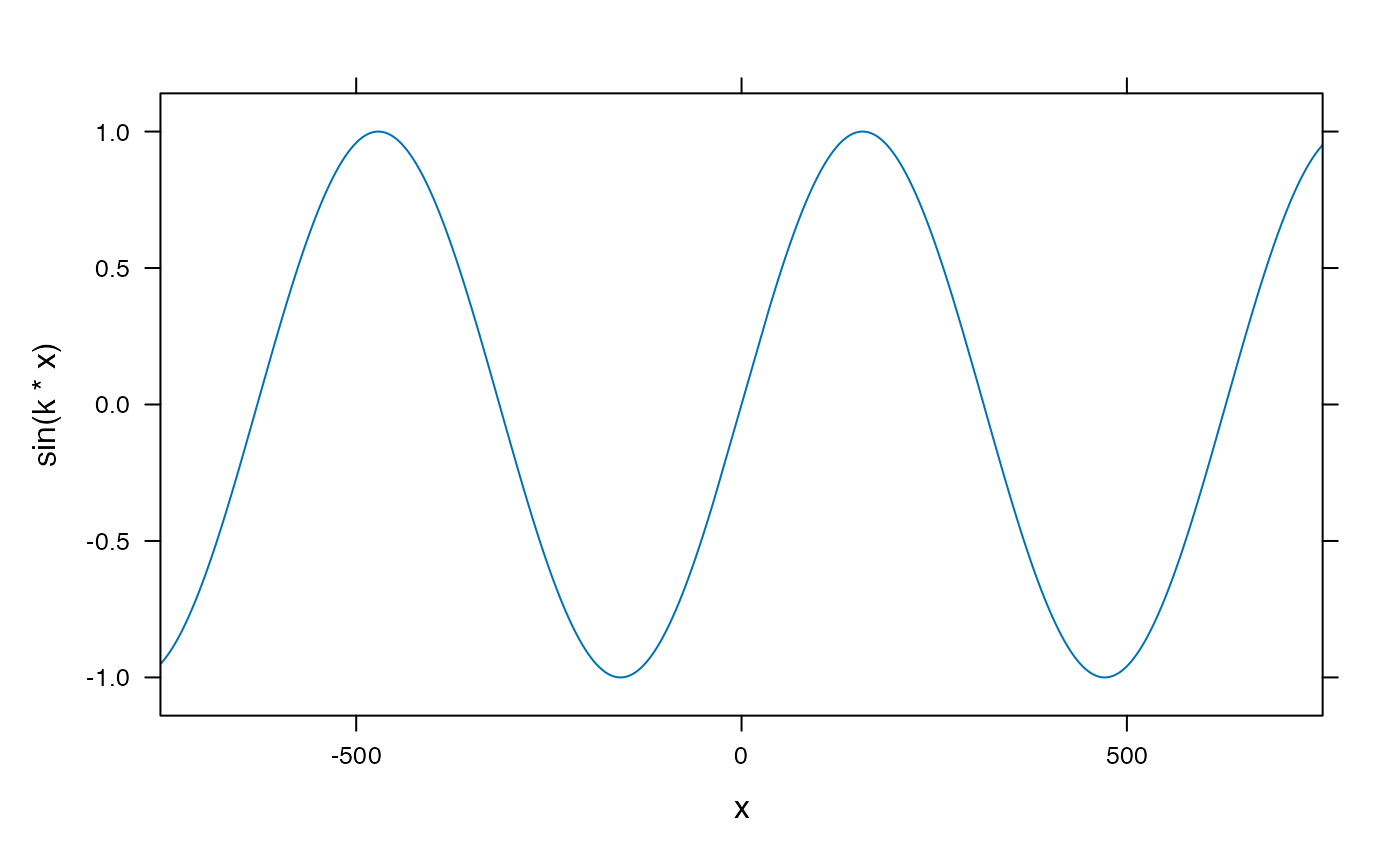# Plotting a linear model with multiple predictors.
mod <- lm(length ~ width * sex, data=KidsFeet)
fitted.length <- makeFun(mod)
xyplot(length ~ width, groups=sex, data=KidsFeet, auto.key=TRUE)plotFun(fitted.length(width, sex="B") ~ width, add=TRUE, col=1)
#> converting numerical color value into a color using lattice settings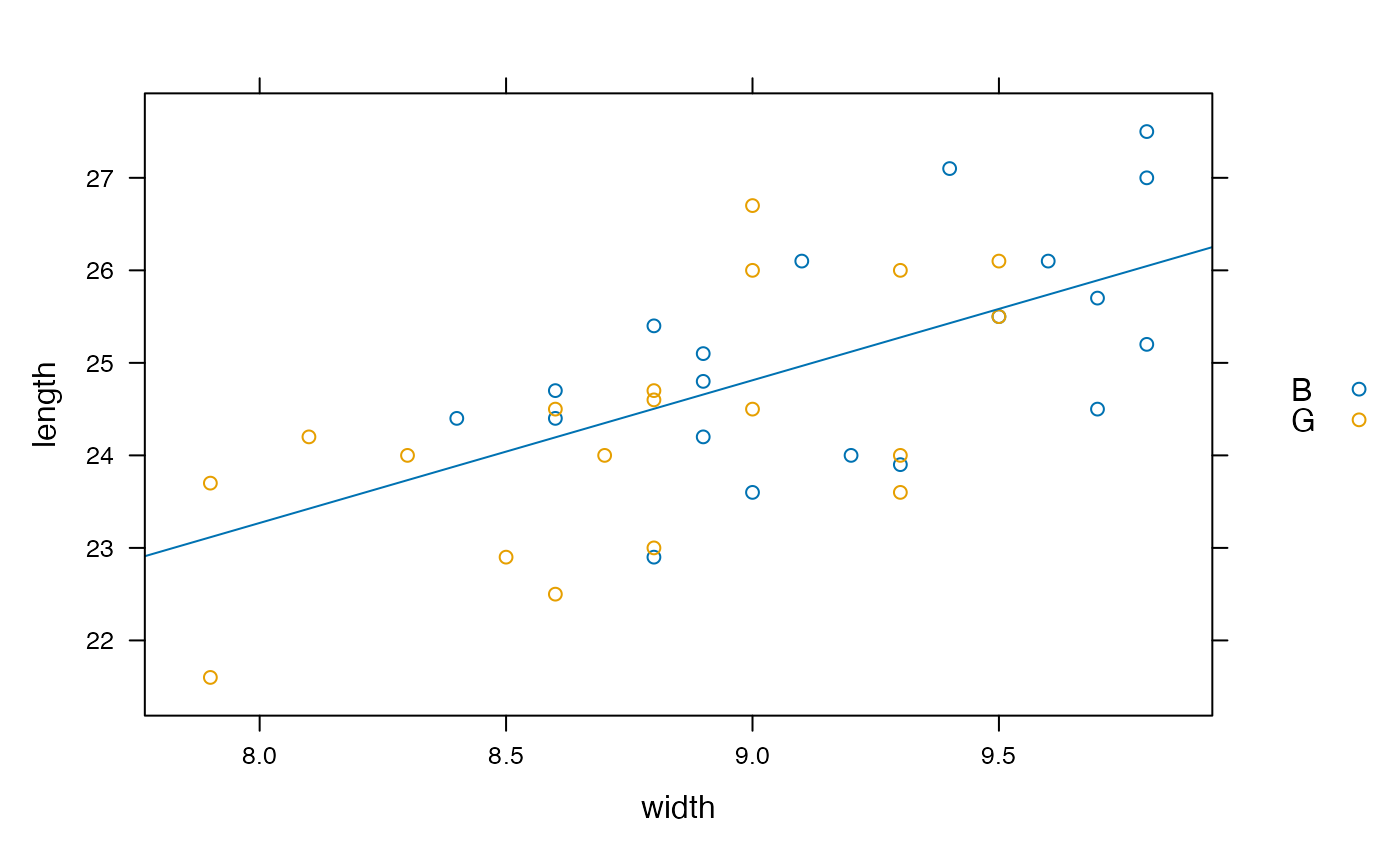plotFun(fitted.length(width, sex="G") ~ width, add=TRUE, col=2)
#> converting numerical color value into a color using lattice settings
#> converting numerical color value into a color using lattice settings